##Rectangular hyperbola relation (line perspectivity)

The figure displays a basic geometric property of the rectangular hyperbola. It represents a relation:
(x-a)*(y-b) = a*b,
satisfied by the parameters x, y of two points X, Y intersected on two lines e1, e2 respectively, by a variable line
through a fixed point P.
The map Y=f(X) of line e1 onto line e2 is called a line perspectivity and point P is called its perspectivity center.
See the discussion in ProjectiveLine.html for the theoretical background.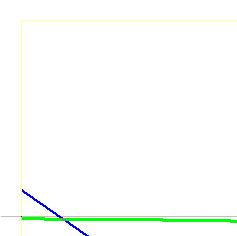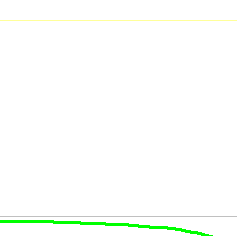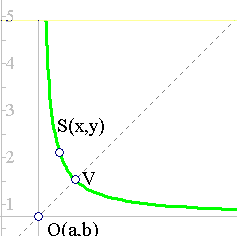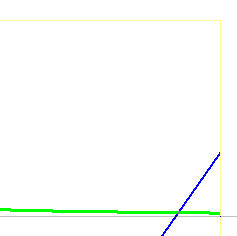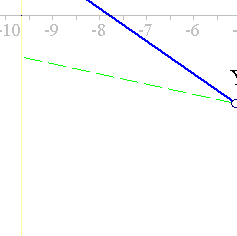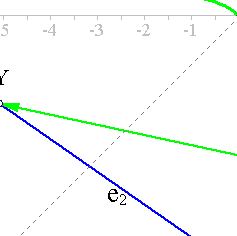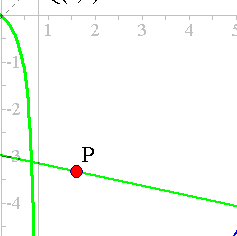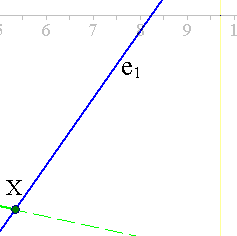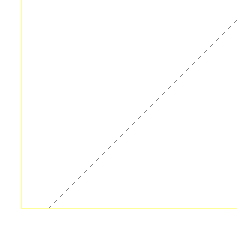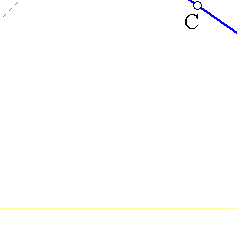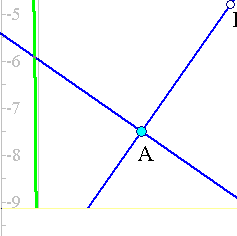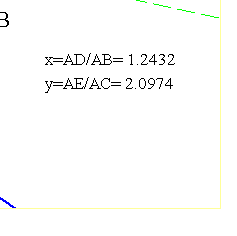This is a particular example of a wide class of maps between the points of two lines, called line projectivities.
The basics for such relations are discussed in the file ProjectiveLine.html and HomographicRelation.html . Points X
and Y=f(X) are supposed to be defined by X = (1-x)*A+x*B and E = (1-y)*A+y*C. The hyperbola depends on the
four points  A, B, C and P. It is constructed in two stages (through user-defined tools of EucliDraw).
Rectangular hyperbolas are discussed in the references below.

Remark This simple fact has many applications and lies on the ground of important theorems, such as
Maclaurin's theorem ( Maclaurin.html ) and Chasles-Steiner theorem ( Chasles_Steiner.html ).